# ChIPS - contouring imagesChIPS Threads (CIAO 4.11 ChIPS v1)

## Overview

#### Synopsis:

This thread is intended to give a simple demonstration of the support for contouring images in ChIPS.

Last Update: 15 Dec 2015 - Updated for CIAO 4.8.

## Introduction

The Starting ChIPS thread describes how to start ChIPS. Please see the ChIPS GUI section of that thread for information on the ChIPS GUI.

The data used in this thread is available in the chips_data.tar.gz file.

unix% ls -1 chips/contour/
a4059_chandra.fits@
a4059_nvss.remap.fits@
chandra.con
nvss.remap.con
projection.dat


The plots used in this thread are based on the data in the images a4059_chandra.fits and a4059_nvss.fits which contain images of the galaxy cluster Abell 4059 from Chandra and the NVSS. Both datasets were taken from the archive, with no re-processing applied. The Chandra image has been binned heavily (by a factor of 16) to increase the signal-to-noise ratio for each pixel. The projection.dat file contains three columns (x axis, y axis, statistic) from a projection run in Sherpa (they are from a spectral analysis of a galaxy cluster, but not Abell 4059).

In CIAO 4.8 the only WCS projection that is supported is the tangent-plane projection. This has meant that the SIN projection used for the NVSS observation of A4059 has been remapped - using the remap tool from the WCSTools package - to create the a4059_nvss.remap.fits file.

The chandra.con and nvss.remap.con files are contour files created by ds9, and were used to create Figure 8.

## Creating a contour plot of an image

In this section we are going to create a contour plot of the a4059_chandra.fits image, and edit the properties of the axes to better display the data. We finish the section by adding a labeled region, used to indicate the size scale of the image.

chips> make_figure("a4059_chandra.fits")
chips> print (info())
Window [win1]
Frame [frm1]
Plot [plot1]   (0.15,0.15)  .. (0.90,0.90)
Border bottom [bx1]  top [bx2]  left [by1]  right [by2]
Contour [ctr1]
X Axis [ax1]
Y Axis [ay1]

chips> print (get_contour().levels)
[500.0, 1000.0, 1500.0, 2000.0, 2500.0]


which uses the make_figure() command to create a contour plot (Figure 1). We will show in later sections how the add_contour() command can also be used.

As we did not specify the contour levels, the command calculated values automatically that span the data range of the image. The levels being displayed can be found by inspecting the levels field of the object returned by get_contour(); in this case there are 5 contours, starting at 500 counts per pixel and extending up to 2500 counts per pixel.

### Figure 1: Basic contour plot of a4059_chandra.fits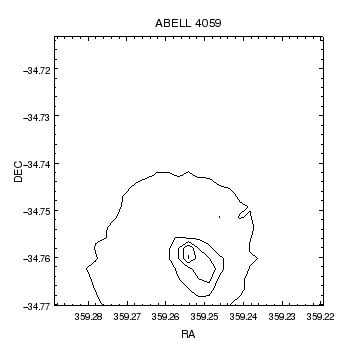### Figure 1: Basic contour plot of a4059_chandra.fits

The make_figure command has created a contour plot of the image data in a4059_chandra.fits. The contour levels were calculated from the image, and the command chose to display the image using the WCS information for the axis ranges (both these defaults can be manually over-ridden).

Since the image is displayed using the WCS information in the file, it is displayed with a fixed aspect ratio; the limits shown in Figure 1 are chosen so as to retain this ratio. Since the default choice does not display all data, we use the panto command to re-center the plot:

chips> get_data_aspect_ratio()
'1:1'
chips> panto(359.255, -34.76)
chips> get_plot_xrange()
[359.28973874149204, 359.22028526399259]
chips> get_plot_yrange()
[-34.788520866501287, -34.731469281718347]


The ChIPS GUI allows you to directly interact with a plot by dragging, panning and zooming the plot limits.

We now customize the plot to improve the labeling of the axes. The resulting figure is shown in Figure 2:

chips> set_xaxis(["tickformat", "ra"])
chips> set_yaxis(["tickformat", "dec"])
chips> set_plot_ylabel("Declination")
chips> yax = ChipsAxis()
chips> yax.ticklabel.angle = 90
chips> yax.ticklabel.offset = 20
chips> yax.ticklabel.halign = 0.5
chips> set_yaxis(yax)


### Figure 2: Creating a publication-quality plot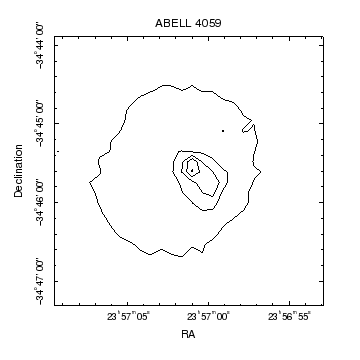### Figure 2: Creating a publication-quality plot

The axes from Figure 1 have been adjusted to produce a publication-quality plot: the numeric labels have been changed from degrees to sexagesimal format, and the ones on the Y axis have been rotated by 90 degrees to avoid overlapping the label and edge of the window.

We now add a region to the plot to act as a scale bar. We choose a width of 30 arcseconds, which corresponds to a maximum radius of 15 * sqrt(2) arcseconds for a square (the remaining factor of 3600 in the add_region call is to convert to degrees).

The radii for WCS axes needs to be corrected by the cos(delta) term, which we apply to the r term when creating the region.

chips> x0 = 359.28
chips> y0 = -34.74
chips> r = 15*np.sqrt(2)/3600
chips> add_region(4, x0, y0, r/np.cos(y0 * np.pi/180))


A label is added to indicate the width of the square. The move_label() command is used to move the label closer to the square (setting the third argument to 1 states that the label should be shifted relative to its old location), and the valign attribute is set to 0.5 to center the label vertically. The result is shown in Figure 3.

chips> add_label(x0-0.01, y0, '30"', ["color", "green"])
chips> move_label(0.002, 0, 1)
chips> get_label().valign
0
chips> set_label(["valign", 0.5, "size", 14])


Note that you can add annotations - such as labels and lines - directly from the GUI, using the Annotate menu item.

### Figure 3: Adding a region and label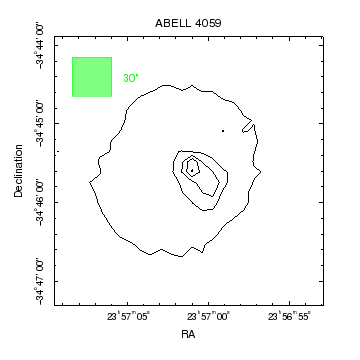### Figure 3: Adding a region and label

The green square has a width of 30 arcseconds.

As previously mentioned, the ChIPS GUI provides support for direct interaction with the visualization. There are also a number of useful commands, such as: zoom, panto, pick_limits, pick and get_pick.

## Overlaying a contour

In this section we shall overlay contours from the file a4059_nvss.remap.fits onto Figure 3.

Our first attempt uses add_contour() to add a second contour plot. The resulting plot, shown in Figure 4, is not useful because the contours chosen for it are not informative.

chips> add_contour("a4059_nvss.remap.fits")


### Figure 4: A first attempt at overlaying radio contours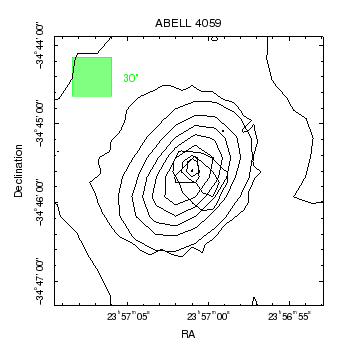### Figure 4: A first attempt at overlaying radio contours

The contour levels chosen for the radio data are:

chips> print(get_contour().levels)
[0.0, 0.1, 0.2, 0.30000000000000004, 0.4, 0.5, 0.6]


We could call undo() and repeat the call, using more sensible contour levels, but here we show how you can change the levels displayed by a contour, using the set_contour() call (the levels were chosen by trial and error based on the range of pixel values in the image):

chips> set_contour(["levels", [0.05, 0.1, 0.3, 0.5]])


The ChIPS GUI can be used to easily change the attributes of objects, including the contour levels, as shown in Figure 5.

### Figure 5: The ChIPS GUI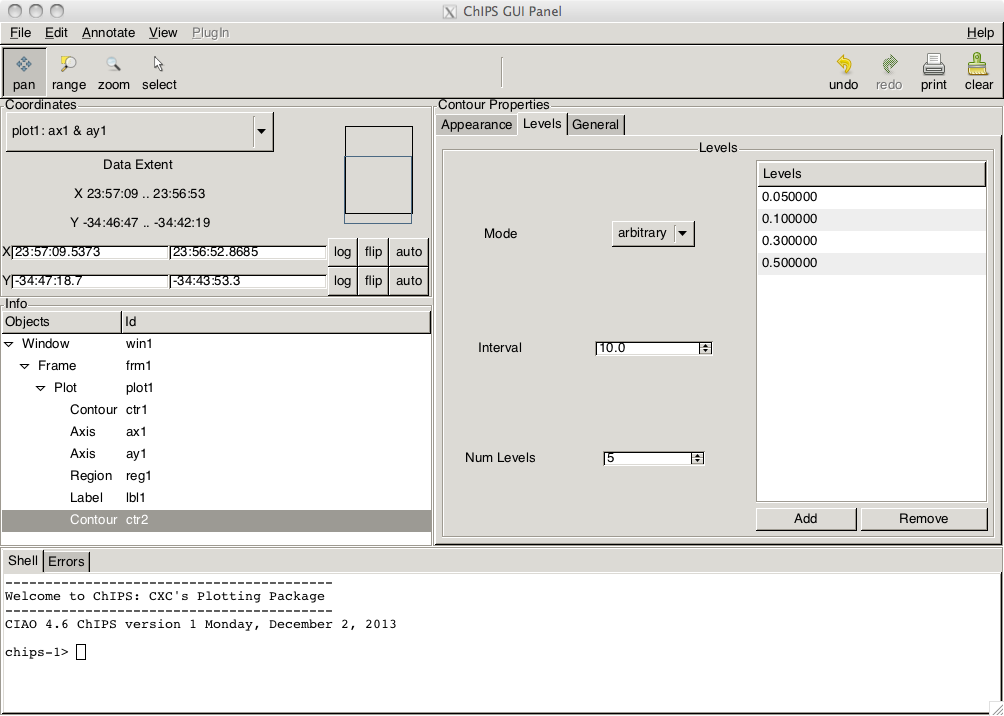### Figure 5: The ChIPS GUI

You can start the ChIPS GUI by either

chips> show_gui()

or by selecting the Show GUI item in the right-mouse-button menu of the ChIPS window.

To clarify the plot contents we decide to change the color of the radio contours to red and add labels to indicate the contour types. The labels are positioned using the "plot-normalized" coordinate system, where (0,0) and (1,1) refer to the bottom-left and top-right corners of the plot (i.e. where the X and Y axes meet).

chips> set_contour(["color", "red"])
chips> add_label(0.95, 0.1, "Chandra", ["coordsys", PLOT_NORM, "halign", 1])
chips> add_label(0.95, 0.15, "NVSS", ["coordsys", PLOT_NORM, "halign", 1, "color", "red"])
chips> save_state("contour-overlay.state")


The save_state() call creates a binary file which can be loaded into another ChIPS session (even on a different machine) by the load_state() command. The result will be the same plot, which can then be edited, changed, or printed.

The resulting figure is shown in Figure 6.

### Figure 6: Cleaning up the plot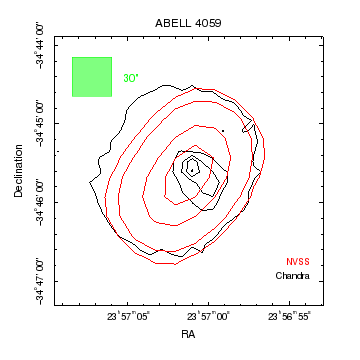### Figure 6: Cleaning up the plot

As the labels have been added using plot-normalized coordinates, they will not move if the axis limits are changed: for example after

chips> limits(Y_AXIS, -35, -34)

the labels remain in the bottom-right of the plot.

chips> undo()

We can also make use of the add_ds9_contours routine from the crates_contrib module, which is part of the CIAO scripts and modules package. First we ensure the code is loaded and then use the routine to display the two contour files saved by ds9:

chips> from chips_contrib.utils import add_ds9_contours
chips> add_ds9_contours('chandra.con', color='green', style='dot', thickness=3)
chips> add_ds9_contours('nvss.remap.con', color='blue', style='dot', thickness=3)
chips> print(info)
Window [win1]
Frame [frm1]
Plot [plot1]   (0.15,0.15)  .. (0.90,0.90)
Border bottom [bx1]  top [bx2]  left [by1]  right [by2]
Contour [ctr1]
X Axis [ax1]
Y Axis [ay1]
Region [reg1]
Label [lbl1]
Contour [ctr2]
Label [lbl2]
Label [lbl3]
Curve [ds9con1]
Curve [ds9con2]



which creates Figure 7. Note that the contour data are actually displayed using curves - with ids of ds9con1 and ds9con2 - and that the routine does not use the standard ChIPS system for setting attributes. To remove the two data sets from the visualization you can either delete them - with two calls to delete_curve - or say

chips> undo(2)

### Figure 7: A direct comparison of ChIPS and ds9 contours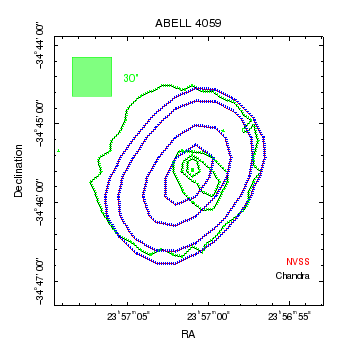### Figure 7: A direct comparison of ChIPS and ds9 contours

The contours from ds9 - green dotted for chandra.con and blue dotted for nvss.remap.con - match those drawn by ChIPS.

The contour plot can also be compared to that created by ds9 -

unix% ds9 a4059_chandra.fits -contour load chandra.con wcs fk5 white \
-contour load nvss.remap.con wcs fk5 red -zoom 16 -cmap b


- shown in Figure 8.

### Figure 8: A ds9 version of the contour plot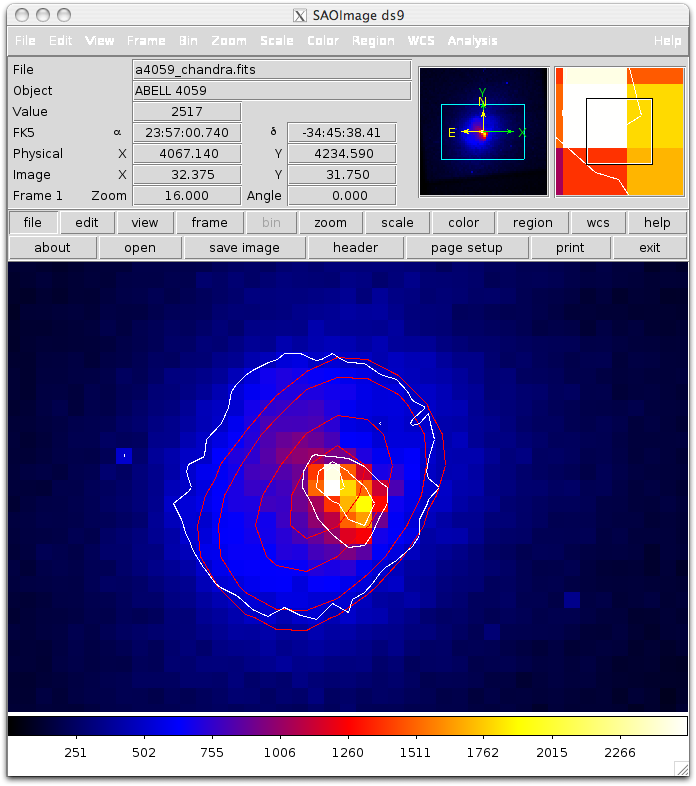### Figure 8: A ds9 version of the contour plot

The white contours are for the X-ray emission, the red contours are the radio emission, and the background image is the Chandra image.

The contour levels were manually set to those used to the values used by ChIPS, and the smoothing scale was set to 1. The contours used are available in the ChIPS tar file as chandra.con and nvss.remap.con.

Since ChIPS includes image support we can also add in the Chandra data as an image using the following commands:

chips> print(info())
Window [win1]
Frame [frm1]
Plot [plot1]   (0.15,0.15)  .. (0.90,0.90)
Border bottom [bx1]  top [bx2]  left [by1]  right [by2]
Contour [ctr1]
X Axis [ax1]
Y Axis [ay1]
Region [reg1]
Label [lbl1]
Contour [ctr2]
Label [lbl2]
Label [lbl3]

chips> add_image("a4059_chandra.fits", ["depth", 50])
chips> set_image(["colormap", "hsv"])
chips> set_axis(["majorgrid.visible", True, "majorgrid.color", "gray"])
chips> zoom(0.7)
chips> move_region(0.003, 0, 1)
chips> move_label("lbl1", 0.006, 0, 1)
chips> set_contour("all", ["thickness", 2])
chips> set_contour("ctr1", ["color", "white"])
chips> set_label("lbl2", ["color", "white"])


which creates Figure 9. Although not shown here, the pick and get_pick commands can be very useful when deciding where to move annotations to. Also note that annotations can be dragged around within the visualization to re-position them, or their coordinates changed from within the GUI.

### Figure 9: Adding an image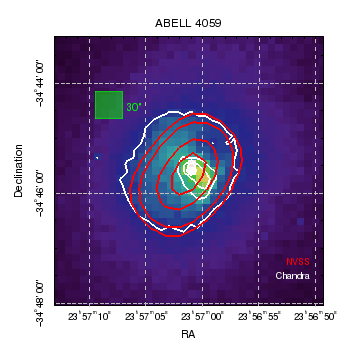### Figure 9: Adding an image

The Chandra data is displayed as an image behind the contours since the image depth is changed to 50 (the contours are at the default depth value of 100). The zoom command has been used to increase the plot range.

Since the annotations used to indicate the image size now overlap the axis grids (displayed since the axis majorgrid.visible attribute was set to True), the label and region are moved slightly using the move_region and move_label commands (as there is only one region we do not need to include an identifier, but we do for the label since there are multiple labels in the visualization). Since both annotations were created using the data coordinate system we use decimal degrees to adjust the location (the third argument being 1 means that we are specifying a relative position in the calls).

In order to make the contours visible we increase the thickness attribute of both contours to 2. The label and contour with a color of default are set to white so that they are visible in the hardcopy output (since the black is not as visible against the image background here).

The ChIPS GUI can be used to easily perform many of these modifications.

## Controlling the contour levels

In this section we shall show how you can change the levels that are used to contour an image, as well as highlight other ways that contours can be created. Note that the ChIPS GUI; can make this task much less of a chore (e.g. Figure 5).

First we read in the image data using the read_file() command, which returns a Crate object representing the most-interesting block in this file (in this case the image). We use this object in the call to add_contour, and specify the levels coordinate system to use.

chips> img = read_file("a4059_chandra.fits")
chips> clear()
chips> levels = [400, 600, 800, 1200, 1800]
chips> add_contour(img, levels, ["wcs", "sky"])
chips> set_plot_xlabel("X")
chips> set_plot_ylabel("Y")


We use the panto command to change the plot display area:

chips> panto(4050, 4250)


### Figure 10: Manually creating a contour plot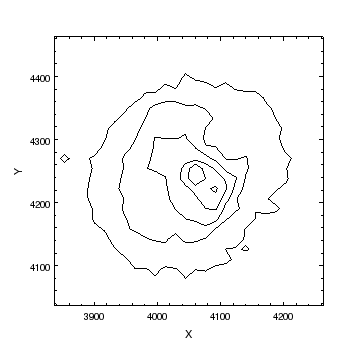### Figure 10: Manually creating a contour plot

This contour was created by manually specifying the contour levels, over-riding the default choice of coordinates (so that the sky coordinates were used rather than the WCS ones), and the data used for the contour was taken from a Crate rather than a file name.

An image array could also have been used, as shown below, but then there would have been no coordinate transform, so the axes would have displayed the logical (i.e. pixel) coordinate system of the array:

chips> ivals = copy_piximgvals(img)
chips> ivals.shape
(64, 65)
chips> add_contour(ivals)


The properties of a contour can be read with the get_contour() command and set with set_contour(). In the following we change the mode field to illustrate different ways that contour levels can be calculated. First we show the contour object:

chips> print(get_contour())
algorithm = 1
color = default
depth = 100
id = None
interval = 10.0
levels = [400.0, 600.0, 800.0, 1200.0, 1800.0]
mode = arbitrary
numlevels = 5
stem = None
style = solid
thickness = 1.0


The mode is set to arbitrary since we manually set the levels when we created the plot. We start by changing the mode to nice, which attempts to use levels at human-readable values:

chips> set_contour(["mode", "nice"])
chips> print (get_contour().levels)
[500.0, 1000.0, 1500.0, 2000.0, 2500.0]


The levels field contains the currently-displayed levels, and can be accessed directly, as shown above. The resulting plot is shown in Figure 11.

### Figure 11: Using the nice mode for contour levels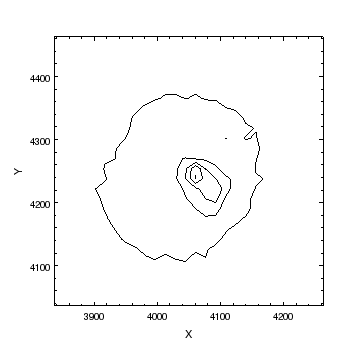### Figure 11: Using the nice mode for contour levels

The nice mode is the default mode for contour plots, so the levels here are the same as those shown in Figure 1. The axes are different since here we explicitly used the SKY coordinate system, rather than the celestial system that is picked by default.

We now set the interval between contours to 200, which implicitly sets the mode to interval, and creates Figure 12.

chips> set_contour(["interval", 200])
chips> get_contour().mode
'interval'
chips> print (get_contour().levels)
[200.0, 400.0, 600.0, 800.0, 1000.0, 1200.0, 1400.0, 1600.0, 1800.0, 2000.0, 2200.0, 2400.0]


### Figure 12: Using the interval mode for contour levels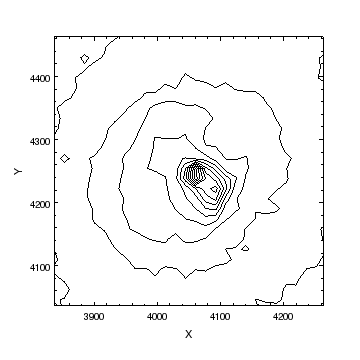### Figure 12: Using the interval mode for contour levels

The interval mode creates levels that are separated by the contour's interval value (here set to 200), starting at the minimum value of the image (in this case 0).

chips> get_piximgvals(img).min()
0


The count mode tries to create a fixed number of levels, given by the numlevels attribute of the contour. The actual number created depends on the data range, as the levels created are meant to be human-friendly in the same way that the nice mode levels are. This is why the following ends up creating a plot with only 2 contours (Figure 13):

chips> set_contour(["numlevels", 4])
chips> get_contour().mode
'count'
chips> print (get_contour().levels)
[1000.0, 2000.0]


### Figure 13: Using the count mode for contour levels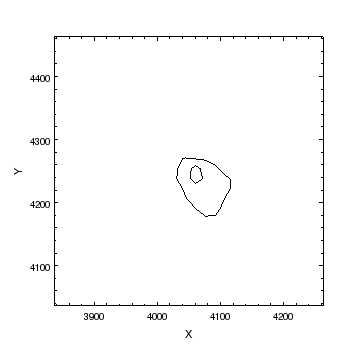### Figure 13: Using the count mode for contour levels

Using the count mode has resulted in a contour with two levels.

Finally we return to the arbitrary mode by specifying the levels to use:

chips> set_contour(["levels", [500, 1000, 1400, 1800, 2200]])
chips> print (get_contour())
algorithm = 1
color = default
depth = 100
id = None
interval = 200.0
levels = [500.0, 1000.0, 1400.0, 1800.0, 2200.0]
mode = arbitrary
numlevels = 4
stem = None
style = solid
thickness = 1.0


The plots in the next section - for example Figure 14 - use these levels.

## Controlling the appearance of a contour plot

We start by by changing the line color and style of the contour plot from the previous section, using a dotted orange line. The result is Figure 14.

chips> set_contour(["color", "orange", "style", "dot"])


### Figure 14: Changing the line style for contours### Figure 14: Changing the line style for contours

The line styles available are the same as for other objects. Due to the way the patterns are constructed, the best results are generally found with the "solid" and "dot" styles.

The results can also depend on the thickness of the contour (here we have used the default value of 1). The on-screen display can look poor when the thickness is set to 0.5 on some graphics cards, but the hard-copy versions created by print_window() are displayed correctly in this case.

We finish by showing how you can create a contour of data in (x,y,z) form - in this case the contents of the text file projection.dat. We start by reading in the data from the file (as the first line of the file is a comment line with three words on it, these words are used as the column names):

chips> !head -5 projection.dat
# kTx Abundance Cash
4.0 1.0e-01 80.36740
4.550 1.0e-01 71.87300
5.100 1.0e-01 66.36260
5.650 1.0e-01 62.58350

chips> cr = read_file("projection.dat")
chips> print (get_col_names(cr))
['kTx', 'Abundance', 'Cash']
chips> kt = copy_colvals(cr, "ktx")
chips> abun = copy_colvals(cr, "abundance")
chips> sval = copy_colvals(cr, "cash")


Since we are about to change the location of the plot in the frame, we ensure that it retains a 1-to-1 plot aspect ratio with the set_plot_aspect_ratio call. We use split to create the second plot, arranged below the first plot; the third argument gives the spacing between the plots. The first plot remains square due to the plot-aspect ratio setting; without it it would have become rectangular. The set_preferences() call makes all future labels default to a size of 14, which saves having to add "size", 14 to the attribute list in the three add_label() calls below.

To identify the contour levels - we use the values returned by Sherpa when the projection was created - we create three contours of the same data, using color and labels to distinguish them. By default, ChIPS will create a name for each new object it makes - such as "plot1" and "cont1" - but this can be over-ridden by setting the id attribute when calling one of the add_<object> calls. We use this to create meaningful names for the contour/label pairs (of 1sig, 2sig, and 3sig).

chips> set_plot_aspect_ratio('1:1')
chips> split(2, 1, 0.1)
chips> set_preferences(["label.size", 14])
chips> add_contour(kt, abun, sval, [53.6454], ["id", "1sig"])
chips> add_label (7, 1, r"1\sigma", ["id", "1sig"])
chips> add_contour(kt, abun, sval, [57.5304], ["id", "2sig", "color", "cyan"])
chips> add_label (9.5, 1.3, r"2\sigma", ["id", "2sig", "color", "cyan"])
chips> add_contour(kt, abun, sval, [63.1794], ["id", "3sig", "color", "brown"])
chips> add_label (11.5, 1.5, r"3\sigma", ["id", "3sig", "color", "brown"])
chips> set_plot_xlabel("kT_x")
chips> set_plot_ylabel("Abundance")


The info command shows the full list of ChIPS objects that have been created in this visualization. We then change to the first plot, using the current_plot command, and add a title to it. If we had not changed plot then the title would have appeared above the second plot and overlap the "X" axis label from the first plot.

chips> print(info())
Window [win1]
Frame [frm1]
Plot [plot1]   (0.15,0.57)  .. (0.90,0.90)
Border bottom [bx1]  top [bx2]  left [by1]  right [by2]
Contour [ctr1]
X Axis [ax1]
Y Axis [ay1]
Plot [plot2]   (0.15,0.15)  .. (0.90,0.48)
Border bottom [bx1]  top [bx2]  left [by1]  right [by2]
Contour [1sig]
X Axis [ax1]
Y Axis [ay1]
Label [1sig]
Contour [2sig]
Label [2sig]
Contour [3sig]
Label [3sig]

chips> current_plot("plot1")
chips> set_plot_title("Multiple contours")
chips> print_window("mplot", ["fittopage", True])
chips> print_window("mplot.pdf", ["fittopage", True])


The print_window() calls create postscript and PDF versions of the plot; by setting the fittopage attribute to True the plot is expanded to fill the page (the keepaspect attribute has been left at its default value of True so that the aspect ratio of the plot is maintained by this expansion). The final plot is shown in Figure 15.

### Figure 15: Multiple contour plots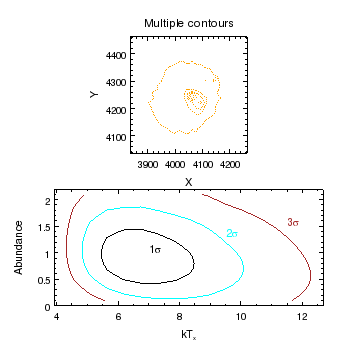### Figure 15: Multiple contour plots

The two plots each contain contoured data of an image; in the top plot there is one contour with multiple levels of a FITS image, and in the second there are three contours (one per level) of data read in from an ASCII file.

The top plot has remained square, even after the call to split, since we set its plot aspect ratio to "1:1".

If we wanted to change the properties of the contours in the second plot, we could use the names we gave as id values to say something like the following (remembering to change the current plot first):

chips> current_plot("plot2")
chips> set_contour("2sig", ["color", "green"])
chips> set_label("2sig", ["color", "green"])


## Summary

• Contours can be created from images in FITS format by sending make_figure() or add_contour() one of:

1. the file name;
2. a crate containing the image, such as the return value of read_file();
3. or an array.

When using add_contour(), contours can also be given as three arrays representing the coordinates (x,y) and value (z) of each pixel. These values can be read from ASCII files.

• The levels at which a contour is drawn can be specified at the time the contour is created, and can be changed afterwards. If no levels are given, then ones will be automatically calculated for you, and there are several different modes for this.

• Contours, as with other ChIPS objects, have a number of attributes that can be read and set using get_contour() and set_contour(). The most useful are the line color, style, and thickness.

• Multiple contours can be overlain on the same plot. These can be the same image, contoured at different values, or different images. Since images often come with multiple coordinate systems - such as logical, physical, and world - contours can be created using any of these systems. This allows contours from different observatories to be combined, such as Figure 6, which displays data from Chandra and the VLA.

In CIAO 4.8 the only supported WCS projection is the tangent-plane system. As discussed above the NVSS data has been re-mapped onto the tangent-plane projection to allow it to be used here in the add_contour routine.

• There is support for axes in Right Ascension and Declination; setting the axis tickformat to ra or dec will use sexagesimal format - rather than decimal degrees - for displaying the axis labels. When using the limits() command to change the axis range being displayed, decimal degrees are always used.

• The aspect ratio of a plot can be controlled; this is automatically set for contours. The get_data_aspect_ratio and set_data_aspect_ratio commands can be used to read and change the current setting.

• The pick() and get_pick() commands can be used to interactively select points from a plot, whether it displays a curve, histogram, or contour.

The ChIPS GUI includes support for directly interacting and modifying ChIPS visualizations.

The limits of a plot can be found using the get_plot_xrange() and get_plot_yrange() calls. Similarly, the ranges of individual objects can be found using the get_contour_xrange() and get_contour_yrange() calls (there are also versions for curves and histograms).

The limits() command can be used to change the axis range to match that of a given object by using the type,id arguments; for example:

chips> limits(chips_contour, "ctr")

• The undo() and redo() commands allow you to remove or re-apply previously-executed commands. When called with no argument they just affect the previous (or next) command in the history. They can also be called with an integer argument, which gives the number of commands from the history buffer to use, or a string, which acts as a tag or marker (assuming you have previously set up the tag using the set_undo_tag() command).

• Once data has been plotted the default coordinate system for positioning and moving items is the data coordinate system. This can be over-ridden, for instance to position a label at the top right corner of a plot you could say:

chips> add_label(0.95, 0.95, label, ["coord_sys", "plot_norm", "halign", 1, "valign", 1])


ChIPS supports a number of coordinate systems, such as data, plot normalized, and frame normalized.

• ChIPS objects will often be automatically assigned a name, of the form <stem><n> - where stem is taken from the preference settings for the object, or they can be explicitly supplied by the user via the id attribute when the object is created. Using your own names for objects can make it easier to distinguish between multiple objects in complicated visualizations.

• The Crates command read_file() can be used to read in images or tables, be they in FITS format or in one of the ASCII formats recognized by the CIAO Data Model. A number of commands, such as get_col_names(), copy_colvals(), and copy_piximgvals() exist for querying and accessing the data in the crate object.

## History

 03 Apr 2009 New for CIAO 4.1 15 Dec 2009 Updated for CIAO 4.2: noted support for multi-dimensional images where extra dimenstions have a length of 1 (e.g. as is common in radio data), the change from get_imagevals to get_piximgvals, the support for setting the aspect ratio of a plot. 15 Dec 2010 Updated for CIAO 4.3: the NVSS image has been converted to a tangent-plane projection since the SIN projection is not supported in CIAO 4.3; an extra plot (Figure 9) has been added to show how image and contours can be combined; several plots have been re-written to use the new zoom and panto routines; the final plot (Figure 15) has been changed to highlight the use of the plot aspect-ratio setting. 15 Dec 2011 Updated for CIAO 4.4: noted the new ChIPS GUI, including Figure 5; added an example of using add_ds9_contours from the script package (Figure 7). 13 Dec 2012 Updated for CIAO 4.5 04 Dec 2013 Updated for CIAO 4.6: noted the change in how non tangent-plane projection systems are handled. 10 Dec 2014 Updated for CIAO 4.7. 15 Dec 2015 Updated for CIAO 4.8.